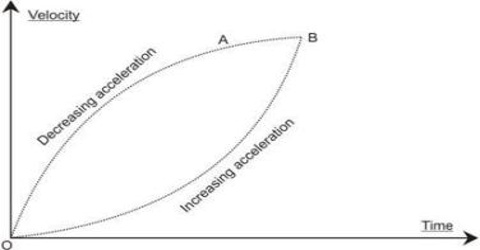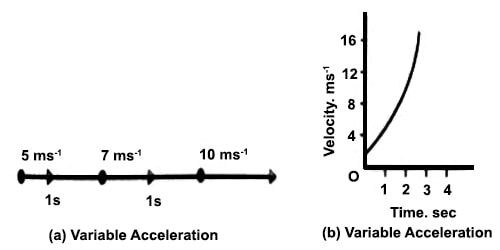Physics

# Variable Acceleration related to MotionVariable acceleration: When the acceleration of a body changes with time, the acceleration is called variable acceleration.

Variable acceleration can be produced due to the change of magnitude and direction or magnitude or direction of acceleration. A body is said to be moving with variable acceleration if its velocity changes by an unequal amount in equal intervals of time, however small these intervals may be. The acceleration of bus, train, car etc. are examples of variable acceleration.In fig. (a) and (b) variable accelerations have been shown by straight line and graph respectively. The slope is different at different points of the graph.

Unit of acceleration: We know, acceleration = (velocity/time). S.I. unit of velocity is metre/sec and that of time is second. So unit of acceleration = (metre/sec)/sec = metre/sec2 = ms-2.

Dimension of acceleration: Acceleration = velocity/time.

Dimensions of velocity are LT-1 and time is T.

So, Dimension of acceleration = [LT-1/T] = [LT-2]